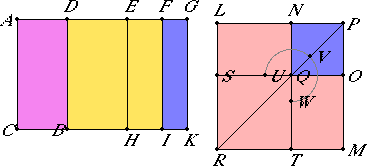# Proposition 92

If an area is contained by a rational straight line and a second apotome, then the side of the area is a first apotome of a medial straight line.

Let the area AB be contained by the rational straight line AC and the second apotome AD.

I say that the side of the area AB is a first apotome of a medial straight line.Let DG be the annex to AD. Then AG and GD are rational straight lines commensurable in square only, and the annex DG is commensurable with the rational straight line AC set out, while the square on the whole AG is greater than the square on the annex GD by the square on a straight line commensurable in length with AG.

X.17

Since the square on AG is greater than the square on GD by the square on a straight line commensurable with AG, therefore, if there is applied to AG a parallelogram equal to the fourth part of the square on GD and deficient by a square figure, then it divides it into commensurable parts.

Bisect, then, DG at E, apply to AG a parallelogram equal to the square on EG and deficient by a square figure, and let it be the rectangle AF by FG. Then AF is commensurable in length with FG.

X.15

Therefore AG is also commensurable in length with each of the straight lines AF and FG.

But AG is rational and incommensurable in length with AC, therefore each of the straight lines AF and FG is also rational and incommensurable in length with AC. Therefore each of the rectangle AI by FK is medial.

X.15

Again, since DE is commensurable with EG, therefore DG is also commensurable with each of the straight lines DE and EG.

But DG is commensurable in length with AC.

X.19

Therefore each of the rectangles DH and EK is rational.

VI.26

Construct the square LM equal to AI, and subtract NO, equal to FK, about the same angle with LM, namely the angle LPM. Then the squares LM and NO are about the same diameter.

Let PR be their diameter, and draw the figure.

Since AI and FK are medial and equal the squares on LP and PN, the squares on LP and PN are also medial, therefore LP and PN are also medial straight lines commensurable in square only.

Since the rectangle AF by FG equals the square on EG, therefore AF is to EG as EG is to FG, while AF is to EG as AI is to EK, and EG is to FG as EK is to FK. Therefore EK is a mean proportional between AI and FK.

But MN is also a mean proportional between the squares LM and NO, and AI equals LM while FK equals NO, therefore MN also equals EK.

But DH equals EK, and LO equals MN, therefore the whole DK equals the gnomon UVW and NO.

Since, then, the whole AK equals LM and NO, and, in these, DK equals the gnomon UVW and NO, therefore the remainder AB equals TS.

But TS is the square on LN, therefore the square on LN equals the area AB. Therefore LN is the side of the area AB.

I say that LN is a first apotome of a medial straight line.

Since EK is rational and equals LO, therefore LO, that is, the rectangle LP by PN, is rational.

But NO was proved medial, therefore LO is incommensurable with NO.

But LO is to NO as LP is to PN, therefore LP and PN are incommensurable in length.

X.74

Therefore LP and PN are medial straight lines commensurable in square only, which contain a rational rectangle. Therefore LN is a first apotome of a medial straight line.

And it is the side of the area AB.

Therefore the side of the area AB is a first apotome of a medial straight line.

Therefore, if an area is contained by a rational straight line and a second apotome, then the side of the area is a first apotome of a medial straight line.

Q.E.D.

## Guide

This proposition is used in X.109.